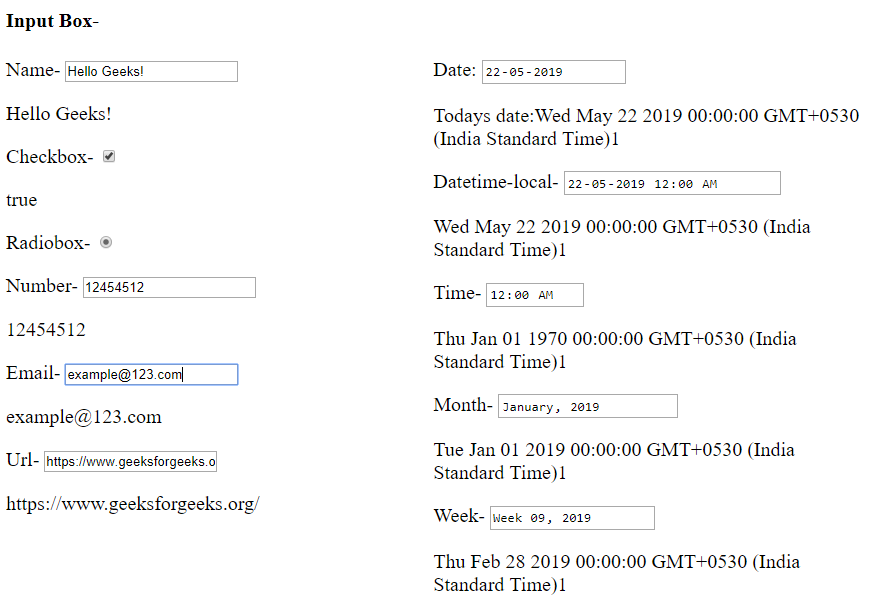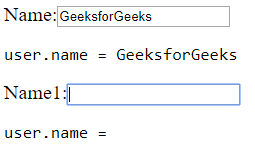Related Articles
AngularJS | ng-model Directive
• Last Updated : 23 May, 2019

ngModel is a directive which binds input, select and textarea, and stores the required user value in a variable and we can use that variable whenever we require that value.
It also is used during validations in a form.

We can use ngModel with:

1. input
• text
• checkbox
• radio
• number
• email
• url
• date
• datetime-local
• time
• month
• week
2. select
3. textarea

Example:

 ```<``html``>``<``script` `src``=``"https://ajax.googleapis.com/ajax/libs/angularjs/1.6.9/angular.min.js"``>````<``style``>``    ``.column {``        ``float: left;``        ``text-align: left;``        ``width: 49%;``    ``}``     ` `    ``.row {``        ``content: "";``        ``display: table;``    ``}```` ` `<``body` `ng-app``=``"myApp"` `      ``ng-controller``=``"myController"``>``    ``<``h4``>Input Box-``    ``<``div` `class``=``"row"``>``        ``<``div` `class``=``"column"``>``            ``Name-``            ``<``input` `type``=``"text"` `                   ``ng-model``=``"name"``>``            ``<``pre``>  {{ name }} Checkbox-``            ``<``input` `type``=``"checkbox"` `                   ``ng-model``=``"check"``>``            ``<``pre``> {{ check }} Radiobox-``            ``<``input` `type``=``"radio"` `                   ``ng-model``=``"choice"``>``            ``<``pre``> {{ choice }} Number-``            ``<``input` `type``=``"number"` `                   ``ng-model``=``"num"``>``            ``<``pre``> {{ num }} Email-``            ``<``input` `type``=``"email"` `                   ``ng-model``=``"mail"``>``            ``<``pre``> {{ mail }} Url-``            ``<``input` `type``=``"url"``                   ``ng-model``=``"url"``>``            ``<``pre``> {{ url }} ``        ````        ``<``div` `class``=``"column"``>``            ``Date:``            ``<``input` `type``=``"date"` `ng-model``=``"date1"` `(change)="log(date1)">``            ``<``pre``> Todays date:{{ date1+1 }} Datetime-local-``            ``<``input` `type``=``"datetime-local"` `ng-model``=``"date2"``>``            ``<``pre``> {{ date2+1 }} Time-``            ``<``input` `type``=``"time"` `ng-model``=``"time1"``>``            ``<``pre``> {{ time1+1 }} Month-``            ``<``input` `type``=``"month"` `ng-model``=``"mon"``>``            ``<``pre``> {{ mon+1 }} Week-``            ``<``input` `type``=``"week"` `ng-model``=``"we"``>``            ``<``pre``> {{ we+1 }} ``        ````    ``````<``script``>``    ``var app = angular.module('myApp', []);``    ``app.controller('myController', function(\$scope) {``        ``\$scope.name = "Hello Geeks!";``        ``\$scope.check = "";``        ``\$scope.rad = "";``        ``\$scope.num = "";``        ``\$scope.mail = "";``        ``\$scope.url = "";``        ``\$scope.date1 = "";``        ``\$scope.date2 = "";``        ``\$scope.time1 = "";``        ``\$scope.mon = "";``        ``\$scope.we = "";``        ``\$scope.choice = "";``        ``\$scope.c = function() {``        ``\$scope.choice = true;``        ``};``    ``});```` ` ``In order to make url and email print, we have to write a valid email/url only then it would get printed. In case of printing of date, time using ngmodel we have to fill in all the fields in the input box. The radio button once selected won’t get deselected since in the function of “c” we are setting the value of choice as true.

Ngmodel using forms:
We can define ngModel in this way also,

Write the below code in app.component.html

 `<``div` `class``=``"form-group"``>``    ``<``label` `for``=``"phone"``>mobile``    ``<``form``>   ``    ``<``input` `          ``type``=``"text"`  `          ``id``=``"phone"` `          ``ngModel ``name``=``"phone"``          ``#``phone``=``"ngModel"``          ``placeholder``=``"Mobile"``>````<``pre``>{{ phone.value }}```

ngModel stores a variable by reference, not value. Usually in binding inputs to models that are objects (e.g. Date) or collections (e.g. arrays).

The phone object created has many fields which are used for validation purpose. We can add the following classes for validation purpose. We are listing only the important ones.

1. ng-touched
2. ng-untouched
3. ng-valid
4. ng-invalid
5. ng-dirty
6. ng-pending
7. ng-pristine

Binding ngModel with getter and setter:
Whenever a function is called with zero arguments then it returns a representation of the model. And when called with a parameter it sets the value. Since ngModel refers to address that is why it does not save the changed value in the object itself rather it saves it in some internal state (variable-name.value). It will be useful if we keep a practice of using getter and setter for models when there is an internal representation as the getter and setter function gets more often called as compared to rest of the parts of the code.

Syntax:

`ng-model-options="{ getterSetter: true }"`

Add this to the input tab.

Example:

 `<``html``>``<``head``>``<``script` `src``=``"https://ajax.googleapis.com/ajax/libs/angularjs/1.6.9/angular.min.js"``>``````<``body` `ng-app``=``"myApp"``>``  ``<``div` `ng-controller``=``"myController"``>``  ``<``form``>``  ``Name:<``input` `type``=``"text"` `name``=``"Name"``             ``ng-model``=``"user.name"``             ``ng-model-options``=``"{ getterSetter: true }"` `/>``  ``<``pre``>user.name = <``span` `ng-bind``=``"user.name()"``>``  ``Name1:<``input` `type``=``"text"` `name``=``"Name1"``             ``ng-model``=``"user.name1"``             ``ng-model-options``=``"{ getterSetter: true }"` `/>``  ``<``pre``>user.name = <``span` `ng-bind``=``"user.name1()"``>``  ```` ````<``script``>``angular.module('myApp', [])``  ``.controller('myController', ['\$scope', function(\$scope) {``    ``name = 'GeeksforGeeks';``    ``name1 = "";``    ``\$scope.user = {``      ``name: function(Name) {``      ``return arguments.length ? (name = Name) : name;``      ``},``      ``name1: function(Name1) {``      ``return arguments.length ? (name1 = Name1) : name1;``      ``} ``    ``};``  ``}]);```````

Here, we have initialized name by the string Geeksforgeeks and name1 by an empty string.Attention reader! Don’t stop learning now. Get hold of all the important DSA concepts with the DSA Self Paced Course at a student-friendly price and become industry ready.

My Personal Notes arrow_drop_up